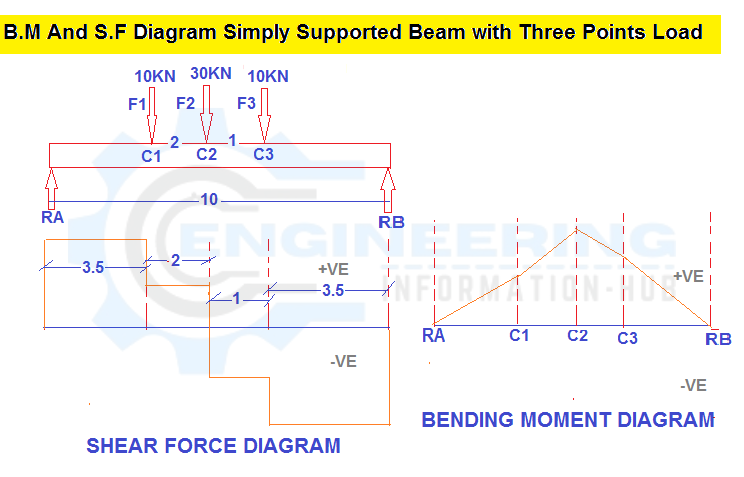Civil Engineering

# B.M And S.F Diagram Simply Supported Beam with Three Points Load

## B.M And S.F Diagram Simply Supported Beam with Three Points Load

Today I will discuss the topic of bending moment and shear force. what is the bending moment | What is the Shear Force |B.M And S.F Diagram Simply Supported Beam with Three Points Load| B.M And S.F Diagram Simply Supported Beam |Simply Supported Beam Three Points Load.

### What is the bending moment?

the bending moment may be a force commonly measured during a force x length (e.g. kNm). Bending moments occur once a force is applied at a given distance off from a degree of reference; inflicting a bending result. within the simplest terms, a bending moment is essentially a force that causes one thing to bend. If the thing isn’t well-restrained the bending force can cause the thing to rotate for a few sure purposes. it would even be value noting you’ll experiment and check out our free calculator to calculate the shear and bending moment diagrams of a beam.

### What is the Shear Force?

Shear force is the force performing on a substance in a direction perpendicular to the extension of the substance, acting in a direction to a flat cross-section of a body.

Shear force is that the summation of the result of shear stress over a surface and infrequently leads to shear strain. Bending moment and shear force calculations ar essential whereas planning any structural members### EXAMPLE

We have the one simply supported beam with the three-point load F1=10kN, F2=30kN, and F3=10kN. The distance between two Reaction Force and span =10m and distance between F1 to F2= 2m and F2 to F3=1m.calculte the bending moment and shear force diagram and calculate the Reaction of RA And R.B.

Given data

F1=C1=10KN

F2=C2=30KN

F3=C3=10KN

The span of the beam =10m

Solution

We know

The sum of upward force = The sum of downward force

R.A+R.B          =             F1+F2+F3

R.A+R.B          =            10+30+10

R.A+R.B            =    50KN———————-     01

Sum of bending moment at A           =     0

R.B x 10-10×6.5-30×5.5-10×3.5=0

10R.B-65-165-35=0

10R.B=65+195+35

10R.B =    265

10 divided by both sides for calculate the value the Rb

10R.B= 265/10

R.B   = 26.5 KN

From equation 01

R.A+R.B            =    50KN

R.A+26.5 =50

R.A= 50-26.5

R.A = 23.5 KN

Now Fist we calculate the shear force

SFB       =      -26.5 KN

SFc3      =      -26.5+10    = -16.5KN

SFc2      =        -16.5+30 = 13.5 KN

SFc1      =         13.5+10   = 23.5KN

SFA= 23.5 KN

Now we calculate the bending moment

BM.b     = 0 KN.M

BM.C3 =    RB X distance (from RB to C3)

BM.C3 =    26.5 x 3.5 = 92.75KN.M

BM.C2 = RB X distance RB to C2 – F3 X distance F3 to C2

BM.C2 =26.5 X 4.5 -10 X 1= 119.25 – 109.25= KN.M

BM.C1 = RB X distance RB to C1– F3 X distance F3 to C1- F2 X distance F2 to C1

BM.C1 =26.5 X 6.5 – 10 X 3 – 30 X 2 = 159 – 30 – 60 = 69 KN.M

BM.A = RB X distance RB to A– F3 X distance F3 to A- F2 X distance F2 to A –F1 X distance F1 to Point A

BM.A = 26.5 X 10 – 10 X 6.5 – 30 X 5.5 – 10 X 3.5 = 265 – 65 -165 – 35= 0

### Raja Numan

Hi, My name is Engr. Raja Numan author of Engineering Information Hub and I am a Civil Engineer by Profession and I've specialized in the field of Quantity Surveying, Land Surveying as QC Engineer in national and multinational companies of Pakistan & Saudi Arabia.

### One Comment

Check Also
Close
error: Content is protected !!